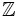Ring of Gaussian integers

This article defines a particular commutative unital ring.
See all particular commutative unital rings

Definition

The ring of Gaussian integers$\mathbb{Z}[i]$ is defined in the following ways:

1. It is the subring generated by the ring of rational integers and the element$i$ (a square root of -1) in the field of complex numbers.
2. It is the integral extension$\mathbb{Z}[t]/(t^2 + 1)$ of the ring of rational integers$\mathbb{Z}$, with the image of the indeterminate$t$ denoted as$i$.
3. It is the ring of integers in the number field$\mathbb{Q}(i)$, a quadratic extension of the rationals given as$\mathbb{Q}[t]/(t^2 + 1)$ (with the image of$t$ denoted$i$).

Ring properties

Property Meaning Satisfied? Explanation
integral domain product of nonzero elements is nonzero Yes Follows from being a subring of$\mathbb{C}$
Euclidean domain has a Euclidean norm Yes In fact, the standard algebraic norm (which in this case is the same as the square of the complex modulus) is a Euclidean norm. The key geometric fact used is that the distance of any point in$\mathbb{C}$ from the closest point in$\mathbb{Z}[i]$ is less than 1.
principal ideal domain integral domain and every ideal in it is a principal ideal Yes See Euclidean implies PID
unique factorization domain every element has a unique factorization into irreducibles up to units Yes See PID implies UFD
Noetherian domain integral domain Yes Follows from being a PID
Bezout domain Yes Follows from being a PID
Dedekind domain Yes Follows from being a PID